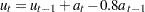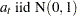## simulating ARIMA transfer Model

I am trying to simulate a transfer model in SAS. I am able to simulate the independent x variable using code provided by sas documentation for a,which is an ARIMA(0,1,1)

``````title1 'Simulated IMA(1,1) Series';
data a;
x1 = 0.9; a1 = 0;
do i = -50 to 100;
a = rannor( 32565 );
x = x1 + a - .8 * a1;
if i > 0 then output;
a1 = a;
x1 = x;
end;
run;``````

but when it comes to simulating the dependent variable y; I start having problems, I have specific values I want for an intercept, numerator, scale and denominator, and including the simulated x variable.i.eWould anyone have any ideas?

Thank You

## Re: simulating ARIMA transfer Model

You used the word ARIMA so I put this in our econometrics board, but maybe this is a more general simulation question.

If so, @Rick_SAS has a large collection of blog posts about simulations, including guidance for targeting specific parameter values.  See Simulate lognormal data with specified mean and variance -- or view the whole series of Simulation articles here.

Discussion stats